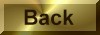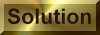Practice Problem 9

Use the value ofGo obtained in Practice Problem 7 to calculate the equilibrium constant for the following reaction at 25°C:

N2(g) + 3 H2(g)2 NH3(g)